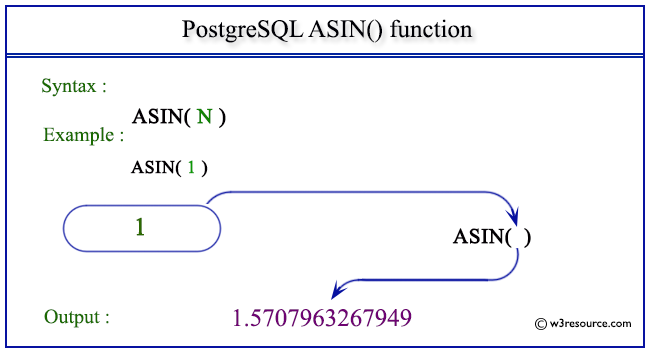# PostgreSQL ASIN() function

## ASIN() function

The PostgreSQL asin() is used to return the inverse sine of a given argument.

Syntax:

```asin(a)
```

PostgreSQL Version: 9.3

Pictorial presentation of PostgreSQL ASIN() functionExample 1: PostgreSQL ASIN() function

Code:

``````SELECT ASIN(0) AS "Asin (0)";
```
```

Sample Output:

``` Asin (0)
----------
0
(1 row)
```

Example 2: PostgreSQL ASIN() function

Code:

``````SELECT ASIN(1) AS "Asin (1)";
```
```

Sample Output:

```    Asin (1)
-----------------
1.5707963267949
(1 row)
```

Example 3: PostgreSQL ASIN() function

Code:

``````SELECT ASIN(-1) AS "Asin (-1)";
```
```

Sample Output:

```    Asin (-1)
------------------
-1.5707963267949
(1 row)
```

Previous: ACOS function
Next: ATAN function

﻿